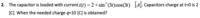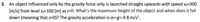# Capacitors Charge & Maximum Height of an object

#### klueless

##### New memberHere are the two of my problems I am having difficulties with. I decided to include both of these into a single thread, because they are both quite basic exercises. Both of these must also be quite simple to solve when I understand some basic principles, but since I am new to differential equations and the teaching hasn't been so good, I have really no clue how to solve these. Help much appreciated!

Thank you guys.

#### Subhotosh Khan

##### Super Moderator
Staff member
View attachment 13460

View attachment 13461

Here are the two of my problems I am having difficulties with. I decided to include both of these into a single thread, because they are both quite basic exercises. Both of these must also be quite simple to solve when I understand some basic principles, but since I am new to differential equations and the teaching hasn't been so good, I have really no clue how to solve these. Help much appreciated!

Thank you guys.
For #2, can you do the following integration:

$$\displaystyle \displaystyle{q(t) \ = \ \int i(t) dt \ = \ \int\left [2 + sin^2(3t)*cos(3t)\right ] dt }$$

You need to post problem#3 in a separate thread. You will first need to derive the expression for the height of the object from the information given. Are you familiar with Galileo's equations for projectile motion?

#### klueless

##### New member
Yes and by integrating i get this:

2t + 1/9 sin^3 (3t) + constant

I will ask more help on #3 in a different thread as you adviced, but let's consider this #2 now for a while.

The thing I'm not seeing is what to do with this integral and what does it actually mean. If it was previously derived, why would I want to get rid of this form where I can actually see the rate of change and what does this integrated form actually show me.

#### Subhotosh Khan

##### Super Moderator
Staff member
Yes and by integrating i get this:

2t + 1/9 sin^3 (3t) + constant

I will ask more help on #3 in a different thread as you adviced, but let's consider this #2 now for a while.

The thing I'm not seeing is what to do with this integral and what does it actually mean. If it was previously derived, why would I want to get rid of this form where I can actually see the rate of change and what does this integrated form actually show me.
What does q(t) mean in this problem?